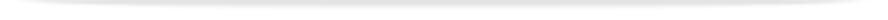units

ENG2091

Faculty of Engineering

This unit entry is for students who completed this unit in 2014 only. For students planning to study the unit, please refer to the unit indexes in the the current edition of the Handbook. If you have any queries contact the managing faculty for your course or area of study.print version

## 6 points, SCA Band 2, 0.125 EFTSL

Refer to the specific census and withdrawal dates for the semester(s) in which this unit is offered, or view unit timetables.

 Level Undergraduate Faculty Faculty of Engineering Offered Clayton First semester 2014 (Day)Malaysia First semester 2014 (Day)Clayton Second semester 2014 (Day) Coordinator(s) Sem 1: Professor Paul Cally (Clayton); Sem 2: Dr Alina Donea (Clayton); Dr Yew Mun Hung (Malaysia)

### Synopsis

Multivariable calculus: double and triple integrals, parametric representation of lines and curves in three dimensional space, use of Cartesian, cylindrical and spherical coordinates, surface and volume integrals, the operations of the gradient, divergence and curl. Ordinary differential equations: solve systems of linear differential equations and the 2nd order Sturm-Liouville type problems. Partial differential equations: the technique of separation of variables and the application of this technique to the wave equation, the heat equation and Laplaces equation.

### Outcomes

On completing this unit, students will be able to represent curves parametrically solve line integrals on these curves; solve double and triple integrals in Cartesian, cylindrical and spherical coordinates; represent surfaces parametrically and solve flux integrals across these surfaces; perform the operations of the gradient, divergence and curl, use these operations in the solution of surface and volume integrals through the Divergence theorem and Stokes theorem; solve systems of simple ordinary differential equations; establish the eigenvalues of these systems; identify and solve 2nd order linear Sturm Liouville differential equations; represent a periodic function with a Fourier series and identify even and odd series expansions; solve elementary partial differential equations through the method of separation of variables; apply this technique to the wave equation, the heat equation and Laplaces equation; classify 2nd order linear partial differential equations as elliptic, parabolic or hyperbolic.

### Assessment

Assignments and test: 30%
Examination (3 hours): 70%

### Chief examiner(s)